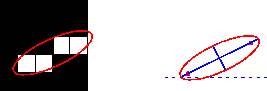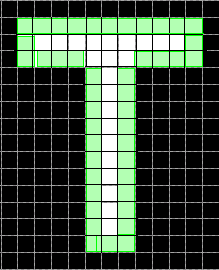# Image Region Analyzer

Browse and filter connected components in an image

## Description

The Image Region Analyzer app measures a set of properties for each connected component (also called an object or region) in a binary image and displays this information in a table. You can also use this app to create other binary images by filtering the image on region properties.

Image Region Analyzer can calculate these properties.

Property NameDescription
`"Area"`Number of pixels in the region.
`"ConvexArea"`Number of pixels in the convex hull. The convex hull is the smallest convex polygon that can contain the region. For more information about classifying pixels on the boundary of the hull, see Classify Pixels That Are Partially Enclosed by ROI.
`"Eccentricity"`Eccentricity of the ellipse that has the same second-moments as the region. The eccentricity is the ratio of the distance between the foci of the ellipse and its major axis length. The value is between 0 and 1. (0 and 1 are degenerate cases. An ellipse whose eccentricity is 0 is actually a circle, while an ellipse whose eccentricity is 1 is a line segment.)
`"EquivDiameter"`Diameter (in pixels) of a circle with the same area as the region, calculated as `sqrt(4*Area/pi)`.
`"EulerNumber"`Euler number (also known as the Euler characteristic), calculated as 1 minus the number of holes in the region.
`"Extent"`Ratio of pixels in the region to pixels in the total bounding box, calculated as `Area` divided by the area of the bounding box.
`"FilledArea"`Number of pixels in the region after filling all holes in the region.
`"MajorAxisLength"`Length (in pixels) of the major axis of the ellipse that has the same normalized second central moments as the region.
`"MinorAxisLength"`Length (in pixels) of the minor axis of the ellipse that has the same normalized second central moments as the region.
`"Orientation"`

Angle (in degrees) between the x-axis and the major axis of the ellipse that has the same second-moments as the region. The value is in the range (–90, 90].

This figure illustrates the axes and orientation of the ellipse. The left side of the figure shows an image region and its corresponding ellipse. The right side shows the same ellipse with the solid blue lines representing the axes. The red dots are the foci. The orientation is the angle between the horizontal dotted line and the major axis.`"Perimeter"`

Distance (in pixels) around the boundary of the region, calculated by adding the distance between each adjoining pair of pixels around the border of the region. This figure illustrates the pixels included in the perimeter calculation for a sample region.`"Solidity"`Proportion of the pixels in the convex hull that are also in the region, calculated as `Area/ConvexArea`.

## Open the Image Region Analyzer App

• MATLAB® Toolstrip: On the Apps tab, under Image Processing and Computer Vision, click the Image Region Analyzer app icon.

• MATLAB command prompt: Enter `imageRegionAnalyzer`.

## Programmatic Use

expand all

`imageRegionAnalyzer` opens the Image Region Analyzer app, which enables you to create other binary images and get information about the regions within binary images.

`imageRegionAnalyzer(I)` opens the Image Region Analyzer app, loading the image `I` into the app.

`imageRegionAnalyzer close` closes all open instances of the Image Region Analyzer app.

## Tips

• Image Region Analyzer uses the `regionprops` function to identify regions in the image and calculate properties of those regions. Note that the `regionprops` offers additional capabilities for calculating region properties:

• Measure properties of images with discontiguous regions

• Measure additional properties such as the centroid and the maximum and minimum Feret diameter and angle

• Measure pixel value properties of grayscale images

• Measure a subset of properties for N-D images

• Perform measurements on a GPU and generate code

• Image Region Analyzer uses the `bwpropfilt` and `bwareafilt` functions to filter binary images.

## Version History

Introduced in R2014b